## Articles tagged "quant"

### GRE Smart Books with Neil: A Mind for Numbers: How to Excel at Math and Science (Even if you Flunked Algebra) by Barbara OakleyCan’t get enough of Neil’s GRE wisdom? Few can. Fortunately, you can join him twice monthly for a free hour and a half study session in Mondays with Neil.

As a long-time instructor of all things standardized testing (GRE, GMAT, LSAT, SAT), I love reading books about math, logic, learning, skill acquisition, neurology, and psychology. Is important to me to stay up-to-date with anything that will help my students use their study time effectively. In this blog series, I’ll be bringing you book reviews and recommendations, as well as excerpts and summaries you can put into practice right away on your GRE journey. Read more

### The Math Beast Challenge Problem of the Week – December 9, 2013Each week, we post a new GRE Challenge Problem for you to attempt. If you submit the correct answer, you will be entered into that week’s drawing for two free Manhattan Prep GRE Strategy Guides.

If x and y are integers such that x < y and xy = 4, which of the following could be the value of 2x + 4y?

To see this week’s answer choices and to submit your pick, visit our Challenge Problem page.

### The Math Beast Challenge Problem of the Week – November 25, 2013Each week, we post a new GRE Challenge Problem for you to attempt. If you submit the correct answer, you will be entered into that week’s drawing for two free Manhattan Prep GRE Strategy Guides.

If n is a positive integer greater than 1, then the function p(n) represents the product of all the prime numbers less than or equal to n. Which of the following is the second smallest prime factor of p(11) + 12?

To see this week’s answer choices and to submit your pick, visit our Challenge Problem page.

### The Math Beast Challenge Problem of the Week – November 11, 2013Each week, we post a new GRE Challenge Problem for you to attempt. If you submit the correct answer, you will be entered into that week’s drawing for two free Manhattan Prep GRE Strategy Guides.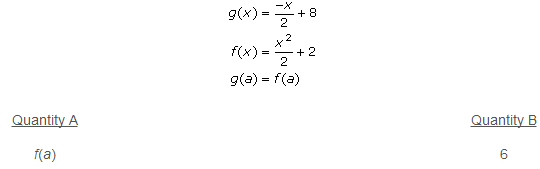• Quantity A is greater.
• Quantity B is greater.
• The two quantities are equal.
• The relationship cannot be determined from the information given.

To see this week’s answer choices and to submit your pick, visit our Challenge Problem page.

### Manhattan GRE’s Halloween Challenge Problem

We hope everyone had a happy Halloween! Yesterday we asked our friends on our Manhattan GRE Facebook page to attempt this Trick-or-Treat Halloween Challenge Problem. As promised, today we are sharing the answer and explanation to the problem: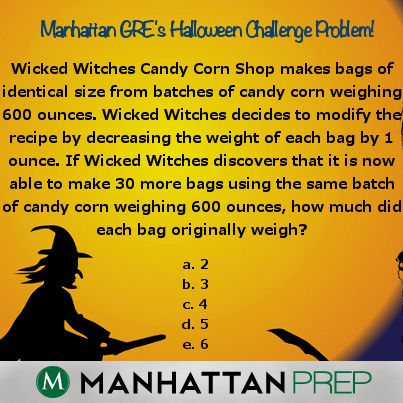Let’s use x for the number of bags produced by the original recipe, and for the weight of each of the bags. Given those variables, our first equation is simply xy = 600. We also need to create an equation that represents the new recipe. Since the number of bags produced has increased by 30, and the weight of each bag has decreased by 1, the new equation is (x + 30)(y – 1) = 600. Remember, the total weight is still 600 ounces. Foiling this equation yields xy – x + 30y – 30 = 600.

We now have two equations with two variables. There are several different paths we can go down here, but all involve substitution of one of the variables, and all will yield a quadratic. The simplest path is to recognize that since xy = 600, we can substitute for xy in the second equation to get 600 – x + 30y – 30 = 600. Subtracting the 600 from both sides, and adding an x to each side gives us 30y – 30 = x. We can now substitute for x in the first equation.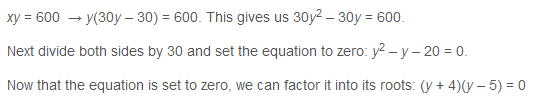### The Math Beast Challenge Problem of the Week – October 28, 2013Each week, we post a new GRE Challenge Problem for you to attempt. If you submit the correct answer, you will be entered into that week’s drawing for two free Manhattan Prep GRE Strategy Guides.

If x is a positive integer and the first nonzero digit in the decimal expansion of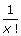is in the hundredths place, what is the value of x?

Submit your pick over on our Challenge Problem page.

### The Math Beast Challenge Problem of the Week – October 21, 2013Each week, we post a new GRE Challenge Problem for you to attempt. If you submit the correct answer, you will be entered into that week’s drawing for two free Manhattan Prep GRE Strategy Guides.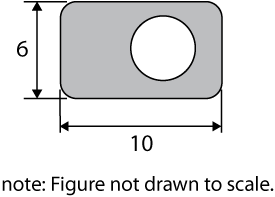The outer figure above is a rectangle with four rounded corners of radius equal to 2.  The inner figure is a circle.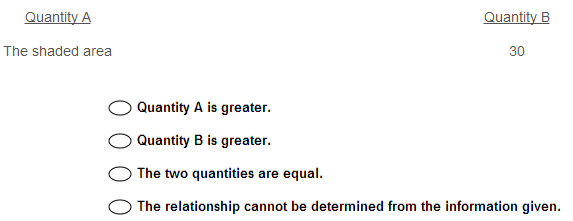Submit your pick over on our Challenge Problem page.

### The Math Beast Challenge Problem of the Week – October 14, 2013Each week, we post a new GRE Challenge Problem for you to attempt. If you submit the correct answer, you will be entered into that week’s drawing for two free Manhattan Prep GRE Strategy Guides.

Xander, Yolanda, and Zelda each have at least one hat. Zelda has more hats than Yolanda, who has more than Xander. Together, the total number of hats the three people have is 12. If Zelda has no more than 5 hats more than Xander, which of the following could be the number of hats that Yolanda has?

See the answer choices and submit your pick over on our Challenge Problem page.

### The Math Beast Challenge Problem of the Week – October 7, 2013Each week, we post a new GRE Challenge Problem for you to attempt. If you submit the correct answer, you will be entered into that week’s drawing for two free Manhattan Prep GRE Strategy Guides.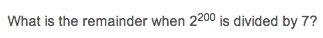See the answer choices and submit your pick over on our Challenge Problem page.

### The Math Beast Challenge Problem of the Week – September 23, 2013Each week, we post a new GRE Challenge Problem for you to attempt. If you submit the correct answer, you will be entered into that week’s drawing for two free Manhattan Prep GRE Strategy Guides.

See the answer choices and submit your pick over on our Challenge Problem page.